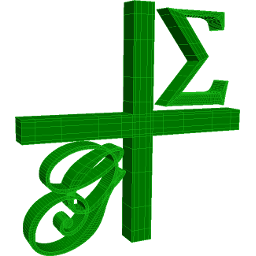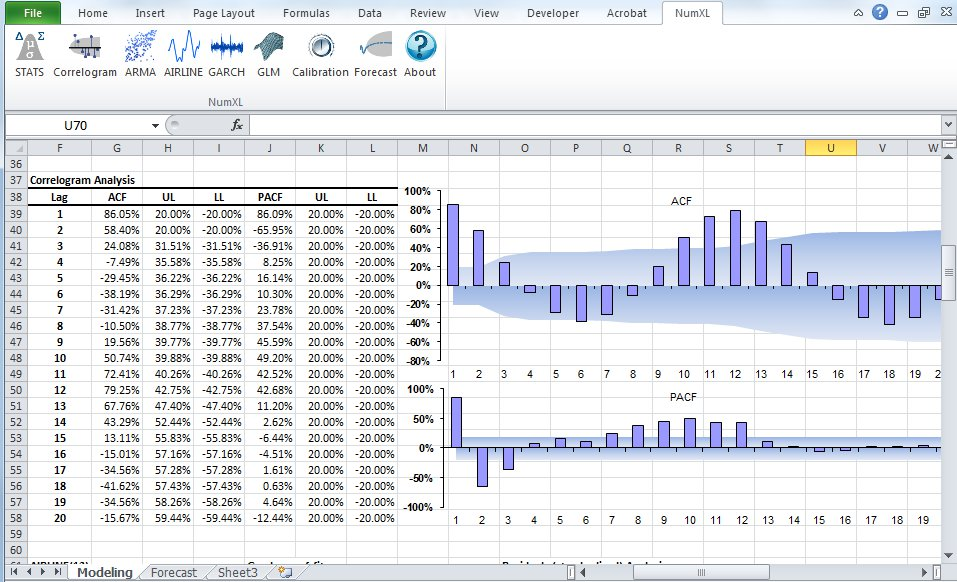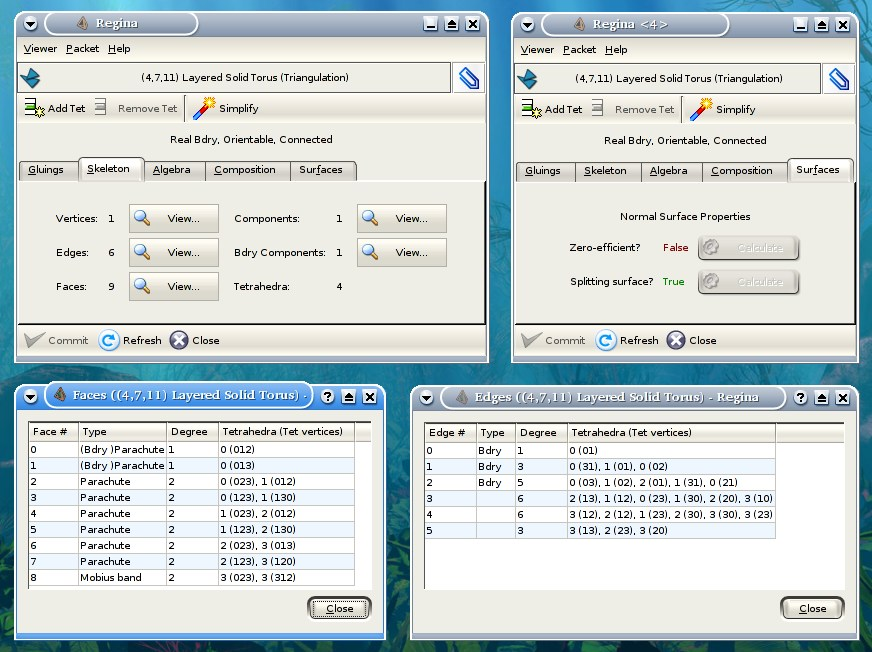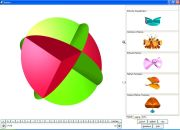## 23 Search Results

The package ADOL-C facilitates the evaluation of first and higher derivatives of vector functions that are defined by computer programs written in C or C++. The resulting derivative evaluation routines may be called from C/C++, Fortran, or any other language that can be linked with C. The numerical values of derivative vectors are obtained free of truncation errors at a small multiple of the run time and randomly accessed memory of the given function evaluation program.

### CGAL

CGAL is a collaborative effort of several sites in Europe and Israel. The goal is to make the most important of the solutions and methods developed in computational geometry available to users in industry and academia in a C++ library. The goal is to provide easy access to useful, reliable geometric algorithms The CGAL library contains: the Kernel with geometric primitives such as points, vectors, lines, predicates for testing things such as relative positions of points, and operations such as intersections and distance calculation, the Basic Library which is a collection of standard data structures and geometric algorithms, such as convex hull in 2D/3D, (Delaunay) triangulation in 2D/3D, planar map, polyhedron, smallest enclosing circle, and multidimensional query structures, the Support Library which offers interfaces to other packages, e.g., for visualisation, and I/O, and other support facilities.

### Fast Artificial Neural Network Library

Fast Artificial Neural Network Library is a neural network library that implements multilayer artificial neural networks in C with support for both fully connected and sparsely connected networks. Cross-platform execution in both fixed and floating point are supported. It includes a framework for easy handling of training data sets. It is easy to use, versatile, well documented, and fast. PHP, C++, .NET, Python, Delphi, Octave, Ruby, Pure Data, and Mathematica bindings are available. A reference manual accompanies the library with examples and recommendations on how to use the library. A graphical user interface is also available for the library.

### G+Smo

G+Smo (Geometry + Simulation Modules, pronounced "gismo") is a new open-source C++ library that brings together mathematical tools for geometric design and numerical simulation. It is developed mainly by researchers and PhD students. It implements the relatively new paradigm of isogeometric analysis, which suggests the use of a unified framework in the design and analysis pipeline. G+Smo is an object-oriented, cross-platform, template C++ library and follows the generic programming principle, with a focus on both efficiency and ease of use. The library is partitioned into smaller entities, called modules. Examples of available modules include the dimension-independent NURBS module, the data fitting and solid segmentation module, the PDE discretization module and the adaptive spline module, based on hierarchical splines of arbitrary dimension and polynomial degree. The library is licenced under the Mozilla Public License v2.0. It has been developed within the homonym research network supported by the Austrian Science Fund and aims at providing access to high quality, open-source software to the forming isogeometric numerical simulation community and beyond.

More information### Givaro

In the joint CNRS-INRIA / INPG-UJF project APACHE, Givaro is a C++ library for arithmetic and algebraic computations. Its main features are implementations of the basic arithmetic of many mathematical entities: Primes fields, Extensions Fields, Finite Fields, Finite Rings, Polynomials, Algebraic numbers, Arbitrary precision integers and rationals (C++ wrappers over gmp) It also provides data-structures and templated classes for the manipulation of basic algebraic objects, such as vectors, matrices (dense, sparse, structured), univariate polynomials (and therefore recursive multivariate). It contains different program modules and is fully compatible with the LinBox linear algebra library and the Athapascan environment, which permits parallel programming.

### GOBLIN Graph Library

A C++ class library including the whole bunch of standard algorithms in graph optimization and drawing. On top of this, a Tcl/Tk wrapper and a GUI for manipulating and editing of graphs.

### HiFlow³

HiFlow³ is a multi-purpose finite element software providing powerful tools for efficient and accurate solution of a wide range of problems modeled by partial differential equations. Based on object-oriented concepts and the full capabilities of C++ the HiFlow³ project follows a modular and generic approach for building efficient parallel numerical solvers. It provides highly capable modules dealing with the mesh setup, finite element spaces, degrees of freedom, linear algebra routines, numerical solvers, and output data for visualization. Parallelism – as the basis for high performance simulations on modern computing systems – is introduced on two levels: coarse-grained parallelism by means of distributed grids and distributed data structures, and fine-grained parallelism by means of platform-optimized linear algebra back-ends.

### igraph

igraph is a collection of network analysis tools with the emphasis on efficiency, portability and ease of use. igraph is a free and open source software package for creating and manipulating undirected and directed graphs. It includes implementations for classic graph theory problems like minimum spanning trees and network flow, and also implements algorithms for some recent network analysis methods, like community structure search. The efficient implementation of igraph allows it to handle graphs with millions of vertices and edges. The rule of thumb is that if your graph fits into the physical memory then igraph can handle it. igraph can be programmed in R, Python and C/C++ by virtue of R/igraph, python-igraph and C/igraph, respectively. There is also a Mathematica interface IGraph/M written by Szabolcs Horvát.

### ILOG CPLEX

ILOG CPLEX is an environment for optimization problems. ILOG CPLEX algorithms can be accessed from the CPLEX Component Libraries as well as the CPLEX Interactive Optimizer, an easy-to-use interactive program. CPLEX provides all the basic features and utilities for using these solvers: sophisticated problem preprocessing; file reading and writing utilities; reporting; messaging control; interactive revision capability; efficient restart from an advanced basis; sensitivity analysis; and an infeasibility finder.

### LattE integrale

LattE (Lattice point Enumeration) is a computer software dedicated to the problems of counting lattice points and integrationinside convex polytopes. LattE contains the first ever implementation of Barvinok's algorithm. The latest version, LattE integrale, has the ability to directly compute integrals of polynomial functions over polytopes and in particular to do exact volume computations. Version 1.6 added the capability of computing the highest coefficients of weighted Ehrhart quasipolynomials.

### LiDIA

LiDIA is a C++ library for computational number theory which provides a collection of highly optimized implementations of various multiprecision data types and time-intensive algorithms.

### LinBox

LinBox is a C++ template library for exact, high-performance linear algebra computation with dense, sparse, and structured matrices over the integers and over finite fields. LinBox has the following top-level functions: solve linear system, matrix rank, determinant, minimal polynomial, characteristic polynomial, Smith normal form and trace. A good collection of finite field and ring implementations is provided, for use with numerous black box matrix storage schemes.

### Normaliz

Normaliz is a tool for computations in affine monoids, vector configurations, lattice polytopes, and rational cones. Its input data can be specified in terms of a system of generators or vertices or a system of linear homogeneous Diophantine equations, inequalities and congruences or a binomial ideal. Normaliz computes the dual cone of a rational cone (in other words, given generators, Normaliz computes the defining hyperplanes, and vice versa), a placing (or lexicographic) triangulation of a vector configuration (resulting in a triangulation of the cone generated by it), the Hilbert basis of a rational cone, the lattice points of a lattice polytope, the normalization of an affine monoid, the Hilbert (or Ehrhart) series and the Hilbert (or Ehrhart) (quasi) polynomial under a Z-grading (for example, for rational polytopes), NEW: generalized (or weighted) Ehrhart series and Lebesgue integrals of polynomials over rational polytopes via NmzIntegrate, a description of the cone and lattice under consideration by a system of inequalities, equations and congruences

### NTL

NTL is a high-performance, portable C++ library providing data structures and algorithms for manipulating signed, arbitrary length integers, and for vectors, matrices, and polynomials over the integers and over finite fields.

### NumXL

NumXL is a suite of time series Excel add-ins. It transforms your Microsoft Excel application into a first-class time series software and econometrics tool, offering the kind of statistical accuracy offered by the leading statistical packages. NumXL integrates natively with Excel, adding scores of econometric functions, a rich set of shortcuts, and intuitive user interfaces to guide you through the entire process.

More information### PolyBoRi

The core of PolyBoRi is a C++ library, which provides high-level data types for Boolean polynomials and monomials, exponent vectors, as well as for the underlying polynomial rings and subsets of the powerset of the Boolean variables. As a unique approach, binary decision diagrams are used as internal storage type for polynomial structures. On top of this C++-library we provide a Python interface. This allows parsing of complex polynomial systems, as well as sophisticated and extendable strategies for Gröbner base computation. PolyBoRi features a powerful reference implementation for Gröbner basis computation.

More information### polymake

polymake is an object-oriented system for experimental discrete mathematics. The typical working cycle of a polymake user starts with the construction of an object of interest, auch as a convex polytope, a finite simplicial complex, a graph, etc. It is then possible to ask the system for some of the object's properties or for some form of visualization. Further steps might include more elaborate constructions based on previously defined objects. Each class of polymake objects comes with a set of rules which describe how a new property of an object can be derived from previously known ones. It is a key feature that the user can extend or modify the set of rules, add further properties or even new classes of objects (with entirely new rule bases). The functions provided include: several convex hull algorithms, face lattices of convex polytopes, Voronoi diagrams and Delaunay decompositions (in arbitrary dimensions), simplicial homology (with integer coefficients), simplicial cup and cap products, intersection forms of triangulated 4-manifolds. Several forms of (interactive) visualization via interfaces to Geomview, JavaView and other programs.

### Regina

Regina is a suite of mathematical software for 3-manifold topologists. It focuses upon the study of 3-manifold triangulations and includes support for normal surfaces and angle structures.

More information### Sage

SAGE is a framework for number theory, algebra, and geometry computation. It is open source and freely available under the terms of the GNU General Public License (GPL). SAGE is a Python library with a customized interpreter. It is written in Python, C++, and C (via Pyrex). Python (http://www.python.org) is an open source object-oriented interpreted language, with a large number of libraries, e.g., for numerical analysis, which are available to users of SAGE. Python can also be accessed in library mode from C/C++ programs. SAGE provides an interface to several important open source libraries, including Cremona’s MWRANK library for computing with elliptic curves, the PARI library (pari.math.u-bordeaux.fr) for number theory, Shoup’s number theory library NTL (http://www.shoup.net/ntl/), SINGULAR (http://www.singular.uni-kl.de) for commutative algebra, GAP (http://www.gap-system.org) for group theory and combinatorics, and maxima (http://maxima.sourceforge.net) for symbolic computation and calculus.

More information### SINGULAR

SINGULAR is a Computer Algebra system for polynomial computations in commutative algebra, algebraic geometry, and singularity theory. SINGULAR's main computational objects are ideals and modules over a large variety of baserings. The baserings are polynomial rings over a field (e.g., finite fields, the rationals, floats, algebraic extensions, transcendental extensions), or localizations thereof, or quotient rings with respect to an ideal. SINGULAR features fast and general implementations for computing Groebner and standard bases, including e.g. Buchberger's algorithm and Mora's Tangent Cone algorithm. Furthermore, it provides polynomial factorizations, resultant, characteristic set and gcd computations, syzygy and free-resolution computations, and many more related functionalities. Based on an easy-to-use interactive shell and a C-like programming language, SINGULAR's internal functionality is augmented and user-extendible by libraries written in the SINGULAR programming language. A general and efficient implementation of communication links allows SINGULAR to make its functionality available to other programs.

### Surfer

Surfer is a programme to visualize real algebraic surfaces in an intuitive and fast way

More information### SYNAPS

SYNAPS (Symbolic and Numeric APplicationS) is a library developed in C++. The aim of this open source project is to provide a coherent and efficient library for symbolic and numeric computation. It implements data-structures and classes for the manipulation of basic objects, such as (dense, sparse, structured) vectors, matrices, univariate and multivariate polynomials. It also provides fundamental methods such as algebraic number manipulation tools, different types of univariate and multivariate polynomial root solvers, resultant computations, ...